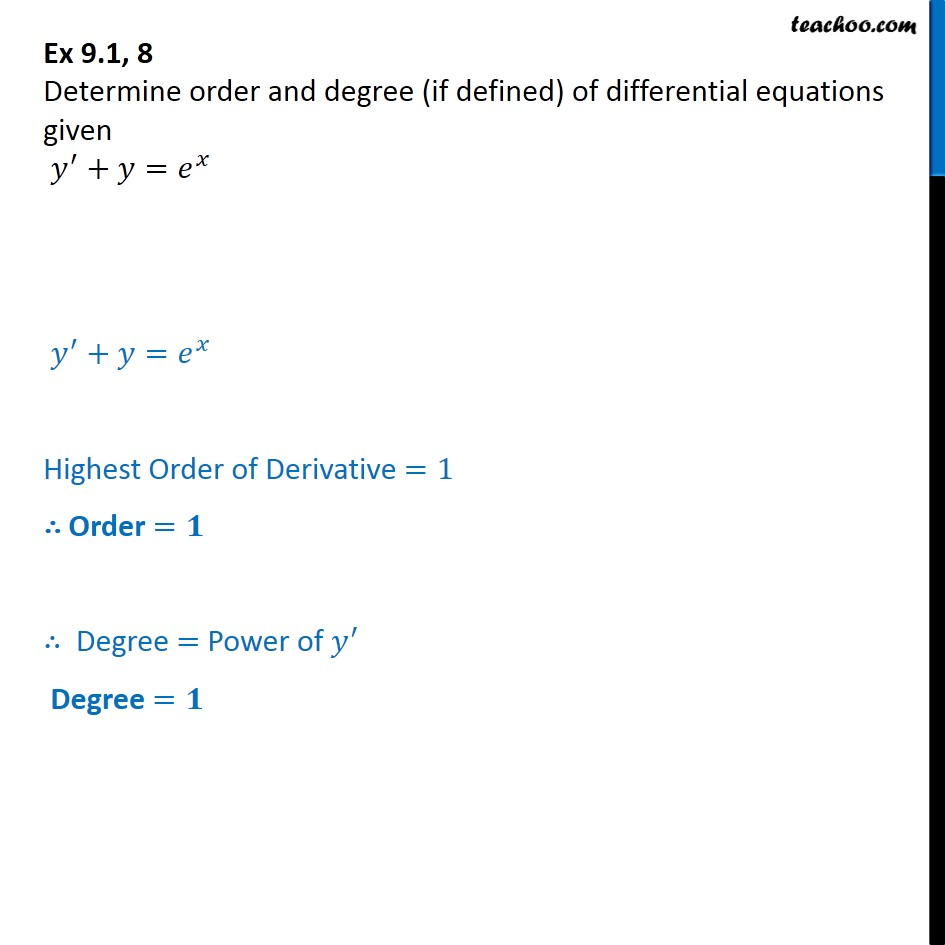Order and Degree

Chapter 9 Class 12 Differential Equations
Concept wiseIntroducing your new favourite teacher - Teachoo Black, at only ₹83 per month

### Transcript

Ex 9.1, 8 Chapter 9 Class 12 Differential Equations NCERT Book Determine order and degree (if defined) of differential equations given y' + y = e^x y' + y = e^x Highest Order of Derivative =1 Order = 1 Degree = Power of y' Degree = 1